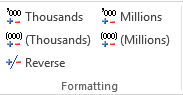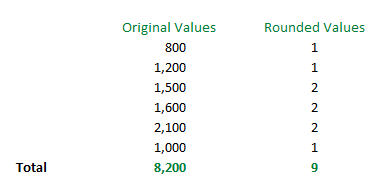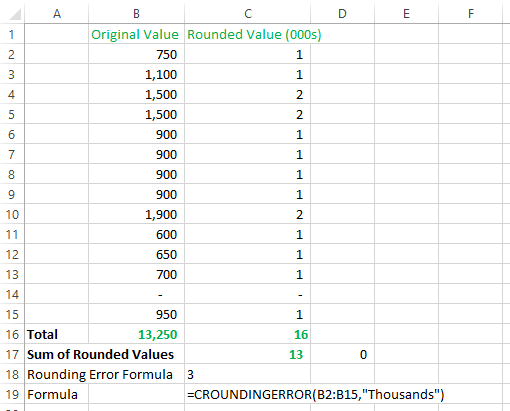# CROUNDINGERROR¶

The CROUNDINGERROR formula is used to calculate the rounding error adjustment for a range of cell values. The cells being calculated must be formatted using the CALUMO format to thousands/millions tool. The return value of this formula is also rounded to the same RoundType as used in the RoundType argument (i.e., thousands/millions).Syntax

CROUNDINGERROR (RoundingRange,RoundType)

### Arguments¶

#### RoundingRange : `string`(required)¶

Specify the rounding range of cell values.

#### Roundtype : `string`(required)¶

Specify the round type that will be applied to cell values

Default value: `"Thousands"`

“Thousands” Rounding in Thousands
“Millions” Rounding in Millions

The RoundType value is flexible in that the following 3 variations will have the same effect: `"Thousands"`, `"thousand"`, `"T"`

## Usage Scenarios¶

To calculate the rounding error adjustment for a range of cell values. The cells being calculated must be formatted using the CALUMO format to thousands functions. The return value of this formula is also rounded to the nearest thousand (or million).

Input of all the arguments is required.

## Examples¶

Assuming the following range of values, which total 8,200. Rounding of this number (to nearest thousand) is 8. When the individual figures are rounded to the nearest thousand, and that value (rounded thousand) is summed, it totals 9. Difference between the two calculations is 1. The CALUMO CROUNDINGERROR function identifies this: